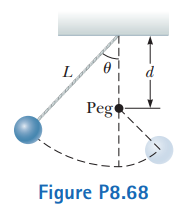# Problem: A pendulum, comprising a light string of length L and a small sphere, swings in the vertical plane. The string hits a peg located a distance d below the point of suspension (Fig. P8.68).(a) Show that if the sphere is released from a height below that of the peg, it will return to this height after the string strikes the peg.(b) Show that if the pendulum is released from rest at the horizontal position (θ = 90°) and is to swing in a complete circle centered on the peg, the minimum value of d must be 3L/5.

###### FREE Expert Solution

This is an energy conservation problem. We'll figure out the energy of the system at various points to derive the required solution.

91% (314 ratings)###### Problem Details

A pendulum, comprising a light string of length L and a small sphere, swings in the vertical plane. The string hits a peg located a distance d below the point of suspension (Fig. P8.68).

(a) Show that if the sphere is released from a height below that of the peg, it will return to this height after the string strikes the peg.
(b) Show that if the pendulum is released from rest at the horizontal position (θ = 90°) and is to swing in a complete circle centered on the peg, the minimum value of d must be 3L/5.Frequently Asked Questions

What scientific concept do you need to know in order to solve this problem?

Our tutors have indicated that to solve this problem you will need to apply the Motion Along Curved Paths concept. You can view video lessons to learn Motion Along Curved Paths. Or if you need more Motion Along Curved Paths practice, you can also practice Motion Along Curved Paths practice problems.

What professor is this problem relevant for?

Based on our data, we think this problem is relevant for Professor Shi's class at USF.

What textbook is this problem found in?

Our data indicates that this problem or a close variation was asked in Physics for Scientists and Engineers - Serway Calc 9th Edition. You can also practice Physics for Scientists and Engineers - Serway Calc 9th Edition practice problems.